Subsections

An SLList (singly-linked list) is a sequence of Nodes. Each node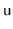stores a data value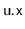and a reference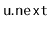to the next node in the sequence. For the last node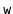in the sequence,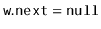```    class Node {
T x;
Node next;
}
```

For efficiency, an SLList uses variables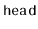and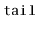to keep track of the first and last node in the sequence, as well as an integer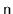to keep track of the length of the sequence:

```    Node head;
Node tail;
int n;
```
A sequence of Stack and Queue operations on an SLList is illustrated in Figure 3.1.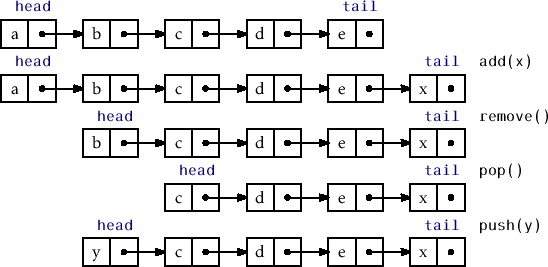An SLList can efficiently implement the Stack operations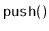and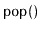by adding and removing elements at the head of the sequence. Theoperation simply creates a new nodewith data value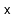, setsto the old head of the list and makesthe new head of the list. Finally, it incrementssince the size of the SLList has increased by one:

```    T push(T x) {
Node u = new Node();
u.x = x;
if (n == 0)
tail = u;
n++;
return x;
}
```

Theoperation, after checking that the SLList is not empty, removes the head by settingand decrementing. A special case occurs when the last element is being removed, in which caseis set to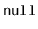:

```    T pop() {
if (n == 0)    return null;
if (--n == 0) tail = null;
return x;
}
```

Clearly, both the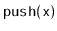andoperations run in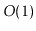time.

## 3.1.1 Queue Operations

An SLList can also implement the FIFO queue operations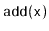and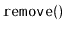in constant time. Removals are done from the head of the list, and are identical to theoperation:

```    T remove() {
if (n == 0)    return null;
if (--n == 0) tail = null;
return x;
}
```

Additions, on the other hand, are done at the tail of the list. In most cases, this is done by setting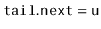, whereis the newly created node that contains. However, a special case occurs when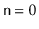, in which case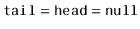. In this case, bothandare set to.

```    boolean add(T x) {
Node u = new Node();
u.x = x;
if (n == 0) {
} else {
tail.next = u;
}
tail = u;
n++;
return true;
}
```

Clearly, bothandtake constant time.

## 3.1.2 Summary

The following theorem summarizes the performance of an SLList:

Theorem 3..1   An SLList implements the Stack and (FIFO) Queue interfaces. The,,andoperations run intime per operation.

An SLList nearly implements the full set of Deque operations. The only missing operation is removing from the tail of an SLList. Removing from the tail of an SLList is difficult because it requires updating the value ofso that it points to the nodethat precedesin the SLList; this is the nodesuch that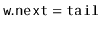. Unfortunately, the only way to get tois by traversing the SLList starting atand takingsteps.

opendatastructures.org